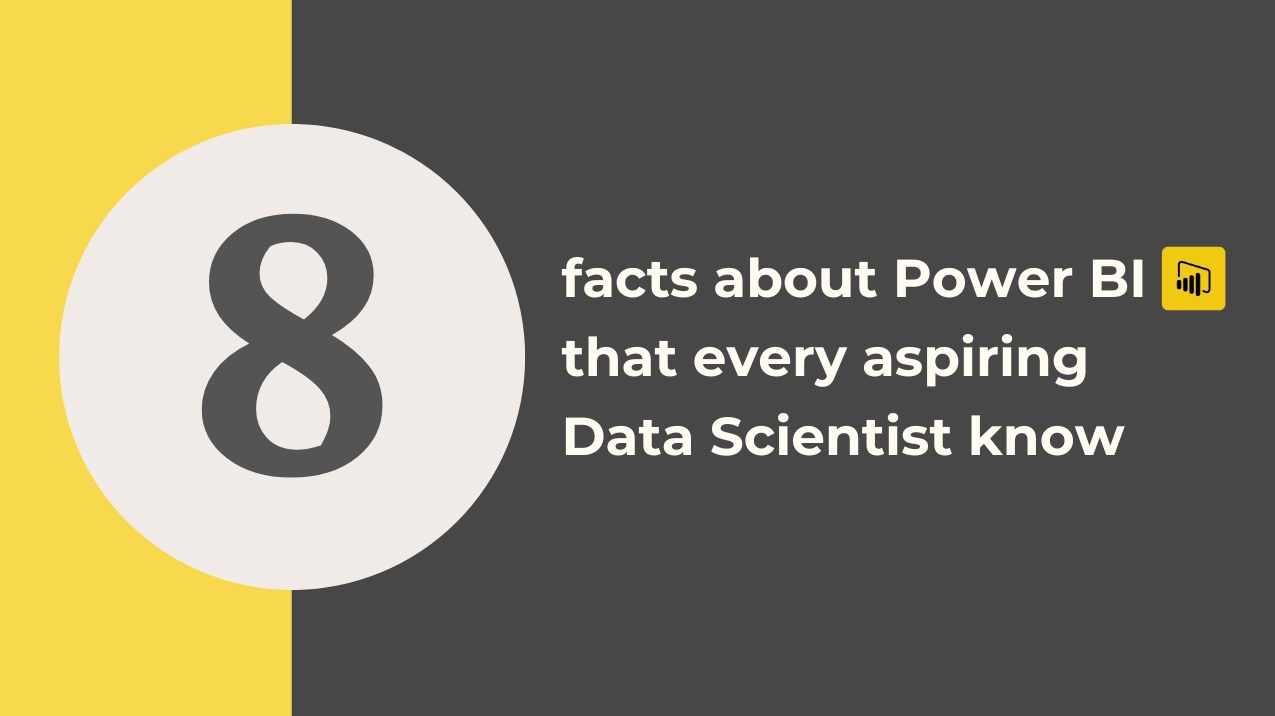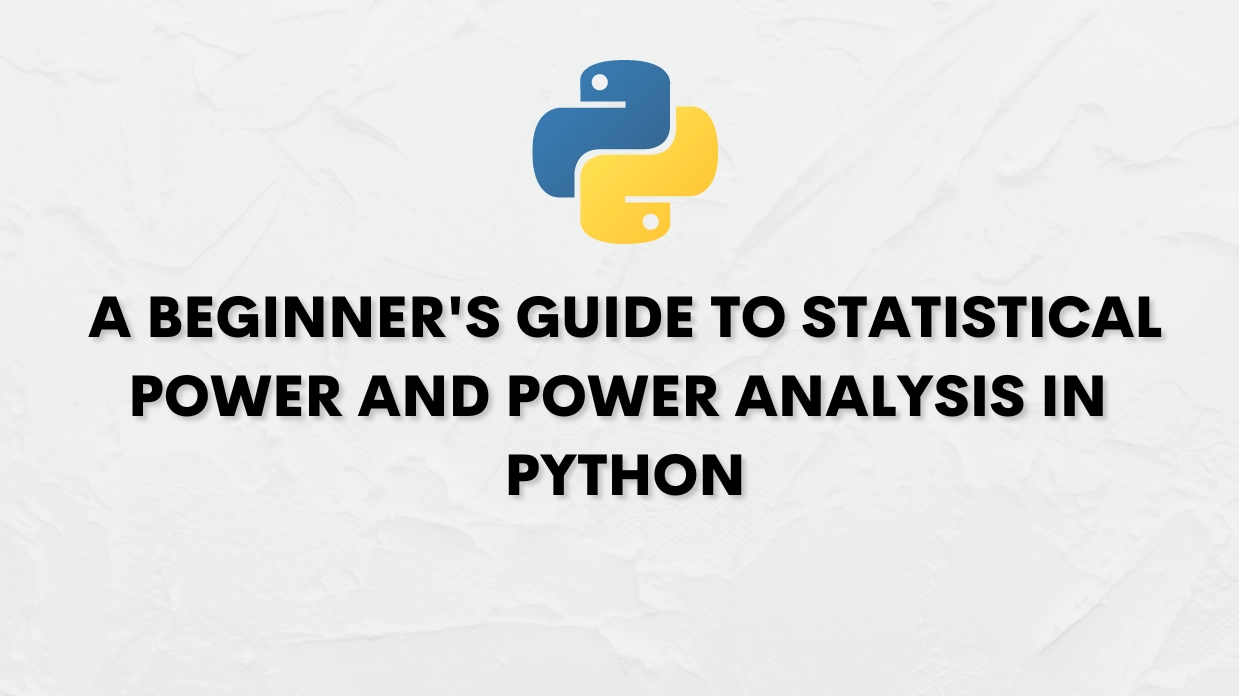#### Recent Post+91 98840 92808# A Beginner's Guide To Statistical Power And Power Analysis In Python

28-Apr-23
• Statistical power is an essential concept in statistics that measures the ability of a statistical test to detect a difference or relationship between two variables when one truly exists. It is the probability of rejecting the null hypothesis when it is false or the probability of making a type II error. Power analysis, on the other hand, is a statistical technique that determines the sample size required to achieve a given level of statistical power for a specific test. In this blog, we will provide a beginner's guide to statistical power and power analysis in Python.

Understanding Statistical Power

Statistical power is influenced by several factors, including the sample size, the significance level, the effect size, and the variability of the data. A larger sample size, a lower significance level, a larger effect size, and a lower variability increase the power of a statistical test. On the other hand, a smaller sample size, a higher significance level, a smaller effect size, and a higher variability decrease the power of a statistical test.

The power of a statistical test is typically denoted by the symbol "1-β" or "γ," where β is the probability of making a type II error and γ is the probability of correctly rejecting the null hypothesis. The power of a statistical test is usually expressed as a percentage, ranging from 0 to 100%.

Calculating Statistical Power in Python

Python has several libraries that can be used to calculate the statistical power of a test. One of the most popular libraries is Statsmodels. Statsmodels is an open-source Python library for statistical modeling that provides a wide range of statistical tests, models, and tools.

To calculate the statistical power of a test using Statsmodels, you first need to import the necessary modules and functions:

import statsmodels.api as sm

from statsmodels.stats.power import TTestIndPower

Next, you need to define the sample size, the significance level, the effect size, and the type of test. For example, let's assume that we want to calculate the statistical power of a two-sample t-test with a sample size of 50, a significance level of 0.05, an effect size of 0.5, and a two-tailed test:

nobs = 50

alpha = 0.05

effect_size = 0.5

power = 0.8

You can then use the TTestIndPower function to calculate the statistical power of the test:

analysis = TTestIndPower()

result = analysis.solve_power(effect_size=effect_size, nobs1=nobs, alpha=alpha, power=power, ratio=1.0, alternative='two-sided')

print(result)

The output will be a float value representing the statistical power of the test.

Understanding Power Analysis

Power analysis is a statistical technique that is used to determine the sample size required to achieve a given level of statistical power for a specific test. The goal of power analysis is to ensure that the sample size is large enough to detect a significant difference or relationship between two variables if one truly exists.

Power analysis involves four key components:

• Significance level: The level of significance is typically set at 0.05 or 0.01, which represents the probability of making a type I error.
• Power: The desired level of statistical power, typically set at 0.80 or 0.90.
• Effect size: The expected size of the effect or difference between the two variables.
• Sample size: The number of observations required to achieve the desired level of statistical power.

Calculating Sample Size for Power Analysis in Python

Python has several libraries that can be used to perform power analysis and calculate the sample size required to achieve a given level of statistical power. One of the most popular libraries for power analysis in Python is Statsmodels, which provides functions for calculating the required sample size for different types of statistical tests, including t-tests, ANOVA, regression analysis, and more.

Our Data Science with Python certification programme is the most in-depth in the business. This Data science programme prepares you for the growing demand for Data science skills and technology in the leading industries.##### ANNA NAGAR :

1St Floor, II Avenue, AC, 3, opp. to Ayyappan Temple, next to Louis Phillippe, Anna Nagar, Chennai, Tamil Nadu 600040